# Addition And Subtraction Of Vectors

Vectors have both magnitude and direction, one cannot simply add two vectors to obtain their sum. The addition of vectors is not as straightforward as the addition of scalars. To better understand this, let us consider an example of a car travelling 10 miles North and 10 miles South. Here, the total distance travelled is 20 miles but the displacement is zero. The North and South displacements are each vector quantities, and the opposite directions cause the individual displacements to cancel each other out. In this article, let us explore ways to carry out the addition and subtraction of vectors.
Table of Content

## Vector Addition:Triangle, Parallelogram and Polygon Law of Vectors

As already discussed, vectors cannot be simply added algebraically. Following are a few points to remember while adding vectors:

• Vectors are added geometrically and not algebraically.
• Vectors whose resultant have to be calculated behave independently of each other.
• Vector Addition is nothing but finding the resultant of a number of vectors acting on a body.
• Vector Addition is commutative. This means that the resultant vector is independent of the order of vectors.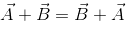### Triangle Law of Vector Addition

The vector addition is done based on the Triangle law. Let us see what triangle law of vector addition is:

Suppose there are two vectors: $$\overrightarrow{a}$$ and $$\overrightarrow{b}$$

Now, draw a line $$AB$$ representing $$\overrightarrow{a}$$ with $$A$$ as the tail and $$B$$ as the head. Draw another line $$BC$$ representing ($$\overrightarrow{b}$$) with $$B$$ as the tail and $$C$$ as the head. Now join the line $$AC$$ with $$A$$ as the tail and $$C$$ as the head. The line $$AC$$ represents the resultant sum of the vectors $$\overrightarrow{a}$$ and $$\overrightarrow{b}$$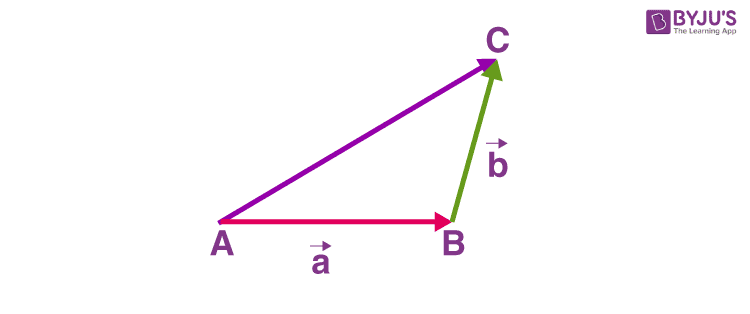The line AC represents $$\overrightarrow{a}$$ + $$\overrightarrow{b}$$

The magnitude of $$\overrightarrow{a}$$ + $$\overrightarrow{b}$$ is:

$$\sqrt{a^2~+~b^2~+~2ab~cos~\theta}$$

Where,

$$a$$ = magnitude of vector $$\overrightarrow{a}$$

$$b$$ = magnitude of vector $$\overrightarrow{b}$$

$$\theta$$ = angle between $$\overrightarrow{a}$$ and $$\overrightarrow{b}$$

Let the resultant make an angle of $$\phi$$ with $$\overrightarrow{a}$$, then:

$$tan\phi$$ = $$\frac{b~sin~\theta}{a~+~b~cos~\theta}$$

Let us understand this by the means of an example. Suppose there are two vectors having equal magnitude $$A$$, and they make an angle $$θ$$ with each other. Now, to find the magnitude and direction of the resultant, we will use the formulas mentioned above.

Let the magnitude of the resultant vector be $$B$$

$$B$$ = $$\sqrt{A^2~+~A^2~+~2AA~cos~\theta}$$ = $$2~A~cos~\frac{θ}{2}$$

Let’s say that the resultant vector makes an angle $$Ɵ$$ with the first vector

$$tan~\phi$$ = $$\frac{A~sin~θ}{A~+~A~cos~θ}$$ = $$tan~\frac{θ}{2}$$

Or,

$$Ɵ$$ = $$\frac{θ}{2}$$

### Parallelogram Law of Vector Addition

The vector addition may also be understood by the law of parallelogram. The law states that “If two vectors acting simultaneously at a point are represented in magnitude and direction by the two sides of a parallelogram drawn from a point, their resultant is given in magnitude and direction by the diagonal of the parallelogram passing through that point.”

According to this law, if two vectors $$\underset{P}{\rightarrow}$$ and $$\underset{Q}{\rightarrow}$$ are represented by two adjacent sides of a parallelogram both pointing outwards as shown in the figure below, then the diagonal drawn through the intersection of the two vectors represent the resultant.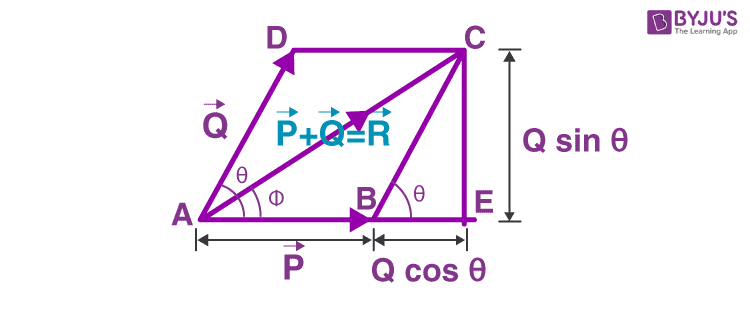In Parallelogram Law of Vector Addition, the magnitude of the resultant is given by: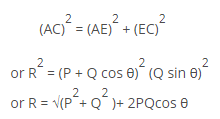The direction of the resultant vector is determined as follows: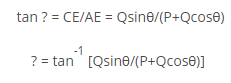### Polygon Law of Vector

According to the polygon law of vector addition, if the number of vectors can be represented in magnitude and direction by the sides of a polygon taken in the same order, then their resultant is represented by magnitude and direction such that the closing side of the polygon is taken in the opposite direction.
Let $$\vec{A}$$, $$\vec{B}$$, $$\vec{C}$$, and $$\vec{D}$$ be the four vectors for which the resultant has to be obtained.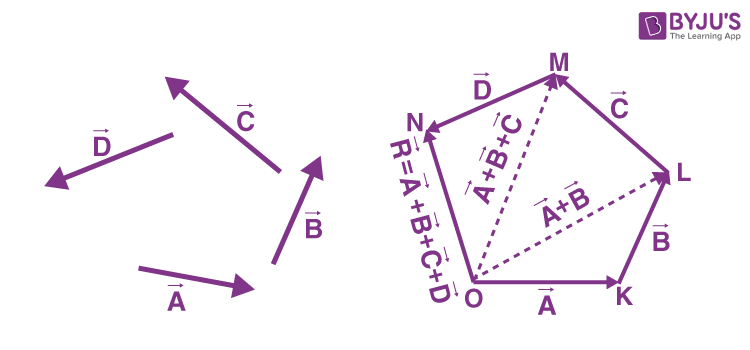Consider triangle OKL, in which the vectors $$\vec{A}$$ and $$\vec{B}$$ are represented by sides $$\vec{OK}$$, $$\vec{KL}$$ and are taken in the same order. Therefore, from triangle law of vector addition we know that the closing side $$\vec{OL}$$ is considered in the opposite direction such that it represents the resultant vector $$\vec{OR}$$ and $$\vec{KL}$$.
Therefore,
$$\vec{OK}$$ + $$\vec{KL}$$=$$\vec{OL}$$ eq.1
From the triangle law of vector addition, we know that triangle OLM can be expressed as $$\vec{OM}$$ is the resultant of the vectors $$\vec{OL}$$ and $$\vec{LM}$$ That is,
$$\vec{OL}$$ + $$\vec{LM}$$=$$\vec{OM}$$ From eq.1,
$$\vec{OK}$$+$$\vec{KL}$$+$$\vec{LM}$$=$$\vec{OM}$$ eq.2
Again, applying the triangle law of vector addition to triangle OMN,
$$\vec{OM}$$+$$\vec{MN}$$$$\vec{ON}$$ From eq.2, we get,
$$\vec{OK}$$+$$\vec{KL}$$$$\vec{LM}$$+$$\vec{MN}$$=$$\vec{ON}$$ eq.3
Therefore,
$$\vec{OK}$$=$$\vec{A}$$ $$\vec{KL}$$, $$\vec{B}$$ $$\vec{LM}$$, $$\vec{C}$$ $$\vec{MN}$$, $$\vec{D}$$ Considering $$\vec{ON}$$=$$\vec{R}$$, the equation becomes
$$\vec{A}$$+$$\vec{B}$$+$$\vec{C}$$+$$\vec{D}$$=$$\vec{R}$$ Click the below video to understand the application of polygon law of vector adding:## Vector Subtraction

Subtraction of two vectors is similar to addition. Suppose $$\overrightarrow{a}$$ is to be subtracted from $$\overrightarrow{b}$$.

$$\overrightarrow{a}$$$$\overrightarrow{b}$$ can be said as the addition of the vectors $$\overrightarrow{a}$$ and (-$$\overrightarrow{b}$$). Thus, the formula for addition can be applied as:

$$\overrightarrow{a}$$$$\overrightarrow{b}$$ = $$\sqrt{a^2~+~b^2~-~2ab~cos~\theta}$$

(-$$\overrightarrow{b}$$) is nothing but $$\overrightarrow{b}$$ reversed in direction.

Related articles:

## Frequently Asked Questions – FAQs

### What is the maximum and minimum sum of two vectors?

The maximum sum of two vectors are obtained when the two vectors are directed in the same direction. The minimum sum is obtained when the two vectors are directed in the opposite direction.

### Is vector addition applicable to any two vectors?

No, vector addition is not applicable to any two vectors. Two vectors are added only when they are of the same types and nature. For instance, two velocity vectors can be added but one velocity vector and one force vector cannot be added.

### State the associative property of vector addition.

The associative property of vector addition states that the sum of the vectors remains the same regardless of the order in which they are arranged.

### Can the sum of two vectors have a zero?

Yes, two vectors with equal magnitude and pointing in opposite directions will have sum equal to zero.